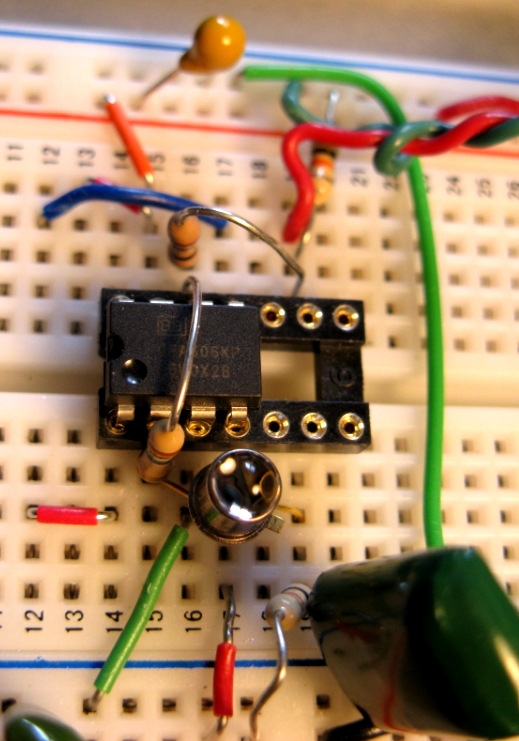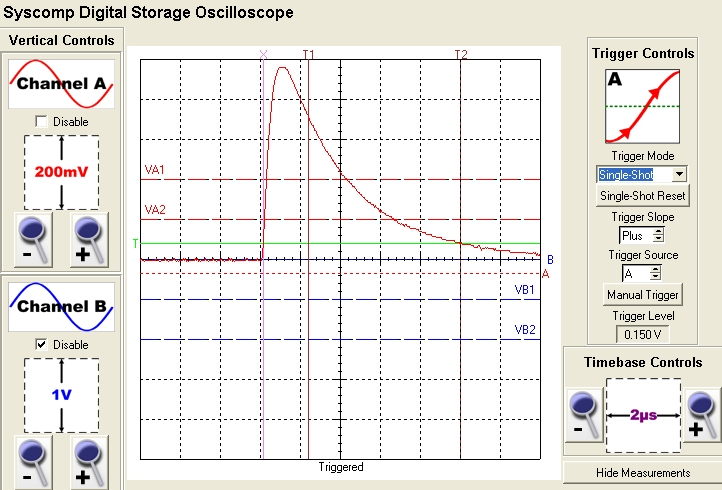# 45 kHz High Gain Transimpedance Photodiode Amplifier

April 12, 2012

The example below demonstrates a high-gain photodiode amplifier implemented with an op-amp transimpedance circuit. The basic transimpedance photodiode amplifier has noise contributions from the feedback resistance thermal noise, the op-amp current-noise spectral density and the op-amp voltage-noise spectral density. The op-amp voltage-noise is amplified in a manner that exhibits a peaking effect. The peaking originates from the noise-gain zero associated with the feedback resistance and the total input capacitance Ci. The amplified op-amp noise-voltage often dominates the total transimpedance output noise as discussed thoroughly in Photodiode Amplifiers, J. Graeme McGraw-Hill, 1996.

The OPA606 op-amp modelled here has a GBW product of 12 MHz, noise spectral densities of en ~ 12 nV/Sqrt(Hz) and in ~ 3 fA/Sqrt(Hz) and fairly low input capacitances (Cicm ~ 3pF, Cid ~ 1 pF). The photodiode is assumed to have a capacitance of 4 pF, typical of a high-speed Si pin photodiode (e.g. Vishay BPW24R at -5V reverse bias). Assuming an extra 2 pf of stray and wiring capacitance, the total input capacitance, Ci is taken as 10 pF. A high transimpedance value of Rf = 10 Mohm is used with a stray shunt capacitance of 0.4 pF across Rf. The basic transimpedance schematic diagram is shown below:The next plot shows the open-loop gain curve |Ao(f)| , transimpedance |Tz(f)| and noise gain |NG(f)| = |1/beta(f)| functions for this op-amp and with the circuit components shown above. The transimpedance 3db bandwidth is ~ 43 kHz and is dominated by the feedback stray capacitance Cf shunting the high feedback resistance of 10 Mohm. This corresponds to a fairly heavily damped response with Q = 0.29 and a considerable phase margin of 85 deg at the intersection point of the noise gain with the open-loop response (at 460 kHz) ensuring stable operation. At low frequencies, the noise-gain is 0 db as expected. The noise-gain zero at 1.5 kHz arising from Rf and the total input capacitance Ci causes the noise-gain to rise until the noise-gain pole at 40 kHz due to Rf and Cf flattens the noise gain curve. The op-amp voltage noise is amplified by this noise-gain profile up to 460 kHz and is then rolled off after this intersection point by the op-amp open-loop response limit. By comparison, the thermal noise of Rf and the op-amp current noise (flowing through Rf) are both rolled-off approximately at the pole frequency 40 kHz. It is obvious that the gain experienced by the op-amp voltage noise contributes extra noise beyond the useful transimpedance signal bandwidth limit of the pole frequency. Note that the Tz(f) rolloff initially at the 3db bandwidth frequency is 20 db/decade, but then changes to 40 db/decade at the frequency of intersection of the noise-gain and open-loop response. This is expected since the transimpedance response with a single-pole op-amp is a 2nd order response circuit and therefore must eventually roll off at this rate:The plots below show the calculated rms cumulative noise terms from each source and the total output noise with increasing frequency. The Rf thermal noise and op-amp current-noise terms level off around the pole frequency of 40 kHz as expected. However the op-amp voltage-noise contribution en continues to increase for an additional decade in frequency until the intersection point of the noise-gain and op-amp open loop response beyond which the noise levels off. This extra region of gain for the op-amp noise voltage is "wasted" gain, since the transimpedance signal gain is rolled off at the much lower pole frequency. This extra "peaking gain" region therefore tends to degrade the S/N ratio. To demonstrate the importance of this, consider the result of using a typical "naive" noise bandwidth for all noise sources as that due to the pole frequency alone. In this simplified noise estimate (which is correct for the Rf thermal noise and the in noise-current contributions only), the effective noise bandwidth would 1.57*Fp = 63 kHz (assuming a single-pole rolloff filter at the pole frequency). This naive estimate suggests a total output noise voltage from all contributors of ~ 110 uV. The true total noise plot below shows that the total output noise voltage is actually ~ 270 uV, almost 2.5 times higher and dominated by the noise-gain peaking experienced by the op-amp voltage-noise.Methods for reducing the effects of the op-amp noise-voltage peaking and thereby reducing overall noise include compensated filtering and post-amp BW filtering of the Tz amp. These modifications are discussed in Graeme's Photodiode Amplifiers.

To verify bandwidth, a OPA606 breadboarded circuit was tested. The stray feedback capacitance across Rf is roughly ~ 0.5 pf for this leaded feedback resistor:A high-speed infrared LED optical impulse of < 30 ns width was used to determine the 3db bandwidth of this photodiode amplifier using the transimpedance response decay time. The scope trace below shows a 90-10% decay time of 7.6 us corresponding to a transimpedance f3db bandwidth of 46 kHz. This corresponds to an actual Cf value of 0.38 pF:An rms total output noise measurement yields ~ 100 uV in a filtered 45 kHz noise bandwidth. This agrees with the cumulative noise plots above and in this filtered measurement is dominated by thermal noise of Rf as expected.